Next: Comparison of pairing models Up: Theoretical framework and results Previous: Theoretical framework and results

## Description of pairing correlations

In our SHF+BCS approach, we use the Skyrme energy density functional in its SLy4 parameterization in the particle-hole channel, whereas two different pairing schemes were implemented in the particle-particle channel. The SHF+BCS(G) scheme employs the seniority pairing force with strength parameters defined as in Ref., i.e.,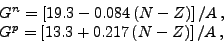(1)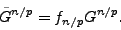(2)

In the SHF+BCS(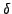) scheme, we apply the state-dependent-interaction with commonly used parameterization variants, which are summarized as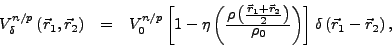(3)

where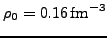and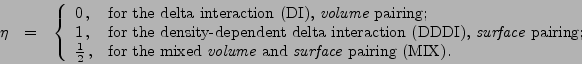(4)

The scaling factors of Eq. (2),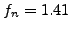and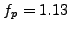, and pairing strengths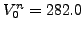MeV,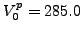MeV (DI),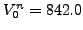MeV,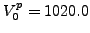MeV (DDDI), and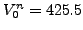MeV,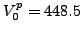MeV (MIX) were adjusted to reproduce the experimental neutron (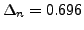MeV) and proton (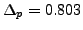MeV) pairing gaps in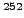Fm. As we deal with contact interactions, we use a finite pairing-active space defined by including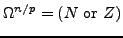lowest single-particle states for neutrons and protons, respectively. In the SHF+BCS() approach, the pairing gap is state dependent. Therefore, the average (spectral) gaps,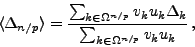(5)

were used as measures of experimental pairing gaps deduced from the odd-even mass staggering. In Eq. (5)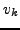and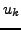are the BCS occupation amplitudes (see, e.g., Ref. for a more detailed discussion).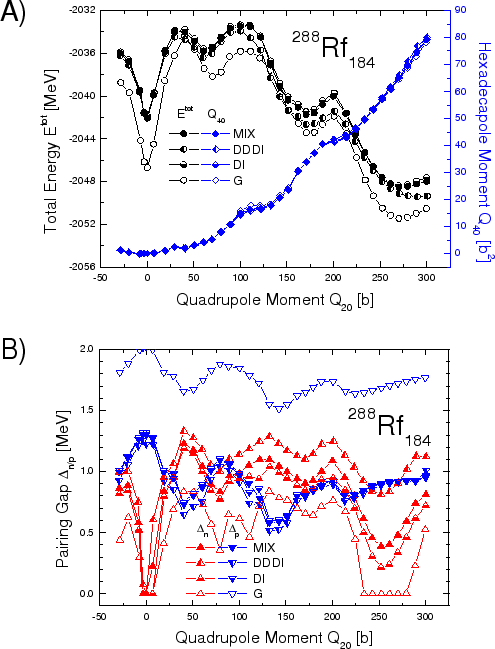The calculations were carried out using the code HFODD (v.2.19l)[17,18,19] that solves self-consistent HF equations by using a Cartesian 3D deformed harmonic-oscillator finite basis. In the calculations, we took the lowest 1140 single-particle states for the basis. This corresponds to 17 oscillator shells at the spherical limit.Next: Comparison of pairing models Up: Theoretical framework and results Previous: Theoretical framework and results
Jacek Dobaczewski 2006-12-10